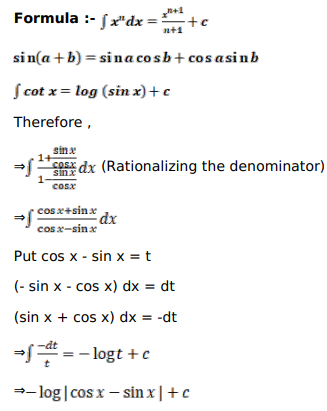# Mark against the correct answer in each of the following:

Question:

Mark $(\sqrt{)}$ ) against the correct answer in each of the following:

$\int \frac{(1+\tan x)}{(1-\tan x)} d x=?$

A. $-\log |\cos x-\sin x|+C$

B. $\log |\cos x-\sin x|+C$

C. $\log |\cos x+\sin x|+C$

D. none of these

Solution: